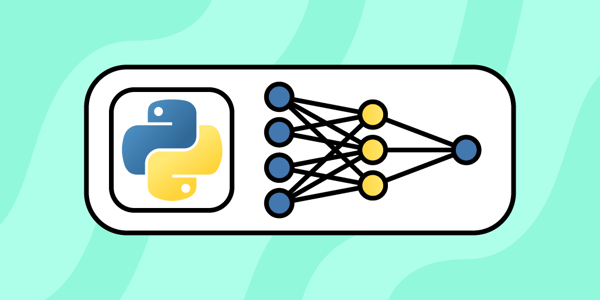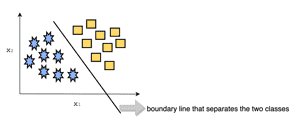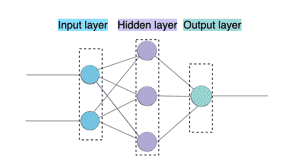May 17, 2022 - 6 min readErica VartanianIf you’ve ever used a voice app like Alexa or Siri, you’ve interacted with a neural network. (And of course, you already have your own neural network – in your brain.)

In machine learning, an artificial neural network (ANN) is an information processing system modeled after our brains. ANNs are the bread and butter of the popular method of advanced machine learning known as deep learning. ANNs are more simply called neural networks, and more recently, deep neural networks.

By learning how to harness neural networks, you can apply them to various interesting use-cases, such as natural-language processing (NLP) and computer vision.

Today we’ll talk about neural networks and how you can start working with them in Python.

We’ll cover:

#### Get hands-on with neural networks today.

Try one of our 300+ courses and learning paths: Become a Deep Learning Professional.

## What are neural networks?

Neural networks are complex structures of machine learning algorithms. A neural network is composed of several information processing units.

The information processing units in ANNs share a name with those in our brains: neurons. However, neurons in an ANN are also called nodes, artificial neurons, or perceptrons.

A perceptron is a machine learning algorithm used for binary classification of data. On its own, a single perceptron constitutes a single-layer neural network, which is the most basic type of neural network.

As a binary classifier, a perceptron can learn linear boundaries between classes, provided it’s given a set of linearly separable training data.

To illustrate, a perceptron uses a linear model to separate a given set of input data into two classes:This is great, but most real-world data isn’t related through a linear relationship.

To perform more complex tasks, neural networks must be able to learn non-linear representations from real-world data. To do this, we need multiple perceptrons to be interconnected in multi-layer neural networks, or multi-layer perceptrons.

Multi-layer neural networks form the basis for deep learning.

When we talk about neural networks, we’re usually referring to multi-layer neural networks.

There are three types of layers in a multi-layer neural network:

• Input layer: Receives a data set of input data, passes inputs to hidden layer
• Hidden layer(s): Contains the main computational units; all computations are performed in these layers
• Output layers: Also contains computational units and returns output data. (There is a debate as to whether the output layer should be considered a hidden layer or not.)

The following figure illustrates a neural network with a single hidden layer:These hidden layers are where the computation happens. Without hidden layers, a neural network will simply return output data identical to the input data.

Hidden layers are what truly enable deep learning.

### Common types of neural networks

While there are various types of neural networks, the most common are:

• Convolutional neural networks: These are commonly used to analyze images, and are the masterminds behind image and facial recognition.
• Recurrent neural networks (RNNs): These learn from sequential training data. They power speech-recognition apps. One type of RNN is the long short-term memory (LSTM) network, which is the type of neural network behind Google Translate.

Neural networks can use different machine learning paradigms, including supervised learning, unsupervised learning, and reinforcement learning.

### How neural networks work

A neural network can receive unstructured data sets, classify data points, recognize patterns, and develop an internal representation through which it makes predictions about similar data sets.

Like humans, a neural network learns to perfect its craft over time. It goes through several iterations of computations and adjustments until it makes predictions to a reasonable accuracy.

Some of the key computational components in neural networks include:

• Activation functions: Each perceptron has an activation function that standardizes its output and prevents different units from collapsing. A common activation function is the sigmoid activation function.
• Weight: A value assigned to connections between perceptrons, estimated by the learning algorithm.

A neural network’s training process looks like this:

• Receives input data: Input data is received through the input layer and passed on to hidden layer(s)
• Generates outputs: The neural network usually does its initial computations by using random numbers as weight assignments
• Compares outputs: The error between the generated output and required output is represented through a loss function.
• Optimizes: An optimization algorithm is used to reduce the loss, an iterative process that repeats until the loss is minimized to a reasonably small value.

Our goal when training neural networks is to reduce the error or loss, which means that the network’s generated outputs will ideally match the required outputs. There are several types of loss functions, a common one being the cross-entropy loss function, which is typical in classification tasks.

To reduce the loss, we update the weights. At this stage, we don’t use random numbers as our weight assignments. Instead, we use optimization algorithms to determine the changes we need to make.

There are many optimization algorithms used to train neural networks. A popular one is the gradient descent algorithm. Gradient descent is an iterative optimization algorithm.

A commonly used variant of gradient descent is stochastic gradient descent, which is well suited for working with large data sets.

## Getting started with neural networks in Python

Creating neural networks (NN) is one of the many amazing things you can do with the Python programming language.

On your way to mastering neural networks, you’ll need a few ingredients:

• Basic Python proficiency
• Deep learning frameworks (such as Keras, TensorFlow, and PyTorch)
• Basic familiarity with linear algebra, probability, and calculus

### Libraries and frameworks for neural networks in Python

There are various libraries and modules you can use to start creating neural networks in Python:

• Keras: Deep learning framework focused on neural networks
• NumPy: Python library packed with high-level mathematical functions for multi-dimensional matrices and arrays
• pandas: Python library for data analysis and data manipulation
• scikit-learn: Python machine learning library for regression and classification
• Matplotlib: Python library for plotting and visualization
• TensorFlow: Machine learning and AI library focused on training neural networks

#### Get hands-on with neural networks today.

Try one of our 300+ courses and learning paths: Become a Deep Learning Professional.

### Neural networks in Python: Code example

Let’s take a sneak peek at how we implement neural networks in Python.

The following Python code is a simple example of image classification using TensorFlow.

# Import packages
import tensorflow as tf
from tensorflow.keras.models import Model
from tensorflow.keras.utils import plot_model
# Build neural network model
def build_model():

# instantiate a Sequential class and linearly stack the layers of your model
seq_model = tf.keras.models.Sequential([tf.keras.layers.Flatten(input_shape=(28, 28)),
tf.keras.layers.Dense(128, activation=tf.nn.relu),
tf.keras.layers.Dense(10, activation=tf.nn.softmax)])
return seq_model
# Instantiate the model and plot it
model = build_model_with_functional()
plot_model(model)
mnist = tf.keras.datasets.fashion_mnist
(training_images, training_labels), (test_images, test_labels) = mnist.load_data()
training_images = training_images / 255.0
test_images = test_images / 255.0
# Set optimization algorithm
loss='sparse_categorical_crossentropy',
metrics=['accuracy'])
# Train and evaluate the model
model.fit(training_images, training_labels, epochs=5)
model.evaluate(test_images, test_labels)

Image classification models learn to recognize images. They’re one of the common types of neural networks we discussed earlier: a convolutional neural network.

## Wrapping up and next steps

There’s more and more data to work with each day. Why not team up with a neural network in Python to do something big with that data?

To help you master neural networks, we’ve created the learning path: Become a Deep Learning Professional. This path covers deep learning fundamentals and includes severals hands-on tutorials and projects to help you master neural networks in Python.

Whether you are:

• A data scientist
• A self-taught innovator looking to change the world (or just someone’s day)
• A person who simply loves to learn (some call us nerds)

… you can harness neural networks to do some amazing things. We can’t wait to see what you do with your own NN.

Happy learning!WRITTEN BYErica Vartanian

Join a community of more than 1 million readers. A free, bi-monthly email with a roundup of Educative's top articles and coding tips.

Learn in-demand tech skills in half the time